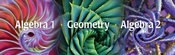Hide other sections

 High School Blog Band Photo Album

WELCOME TO HIGH SCHOOL MATH!HIGH SCHOOL MATH

GEORGIA STANDARDS OF EXCELLENCE FOR HIGH SCHOOL MATH

COURTESY OF: https://www.georgiastandards.org/Georgia-Standards/Documents/High-School-Mathematics-Standards.pdf

Mathematics | High School

The high school standards specify the mathematics that all students should study in order to be college and career ready. The standards are listed in conceptual categories which portray a coherent view of high school mathematics:

• Number and Quantity
• Algebra
• Functions
• Geometry
• Statistics and Probability

Mathematics | High School — Number and Quantity

The Real Number System

• Extend the properties of exponents to rational exponents
• Use properties of rational and irrational numbers.

Quantities

• Reason quantitatively and use units to solve problems

The Complex Number System

• Perform arithmetic operations with complex numbers
• Represent complex numbers and their operations on the complex plane
• Use complex numbers in polynomial identities and equations

Vector and Matrix Quantities

• Represent and model with vector quantities.
• Perform operations on vectors.
• Perform operations on matrices and use matrices in applications.

Mathematics | High School — Algebra

Seeing Structure in Expressions

• Interpret the structure of expressions
• Write expressions in equivalent forms to solve problems

Arithmetic with Polynomials and Rational Expressions

• Perform arithmetic operations on polynomials
• Understand the relationship between zeros and factors of polynomials
• Use polynomial identities to solve problems
• Rewrite rational expressions

Creating Equations

• Create equations that describe numbers or relationships

Reasoning with Equations and Inequalities

• Understand solving equations as a process of reasoning and explain the reasoning
• Solve equations and inequalities in one variable
• Solve systems of equations
• Represent and solve equations and inequalities graphically

Mathematics | High School — Functions

Interpreting Functions

•  Understand the concept of a function and use function notation
• Interpret functions that arise in applications in terms of the context
• Analyze functions using different representations

Building Functions

• Build a function that models a relationship between two quantities
• Build new functions from existing functions

Linear, Quadratic, and Exponential Models

• Construct and compare linear, quadratic, and exponential models and solve problems
• Interpret expressions for functions in terms of the situation they model

Trigonometric Functions

• Extend the domain of trigonometric functions using the unit circle
• Model periodic phenomena with trigonometric functions
• Prove and apply trigonometric identities

Mathematics | High School — Geometry

Congruence

• Experiment with transformations in the plane
• Understand congruence in terms of rigid motions
• Prove geometric theorems
• Make geometric constructions

Similarity, Right Triangles, and Trigonometry

• Understand similarity in terms of similarity transformations
• Prove theorems involving similarity
• Define trigonometric ratios and solve problems involving right triangles
• Apply trigonometry to general triangles

Circles

• Understand and apply theorems about circles
• Find arc lengths and areas of sectors of circles

Expressing Geometric Properties with Equations

• Translate between the geometric description and the equation for a conic section
• Use coordinates to prove simple geometric theorems algebraically

Geometric Measurement and Dimension

• Explain volume formulas and use them to solve problems
• Visualize relationships between two-dimensional and three-dimensional objects

Modeling with Geometry

• Apply geometric concepts in modeling situations

Mathematics | High School — Statistics and Probability

Interpreting Categorical and Quantitative Data

•  Summarize, represent, and interpret data on a single count or measurement variable
• Summarize, represent, and interpret data on two categorical and quantitative variables
• Interpret linear models

Making Inferences and Justifying Conclusions

• Understand and evaluate random processes underlying statistical experiments
• Make inferences and justify conclusions from sample surveys, experiments and observational studies

Conditional Probability and the Rules of Probability

• Understand independence and conditional probability and use them to interpret data
• Use the rules of probability to compute probabilities of compound events in a uniform probability model

Using Probability to Make Decisions

• Calculate expected values and use them to solve problems
• Use probability to evaluate outcomes of decisionsCopyright © 2019  Taliaferro County Schools